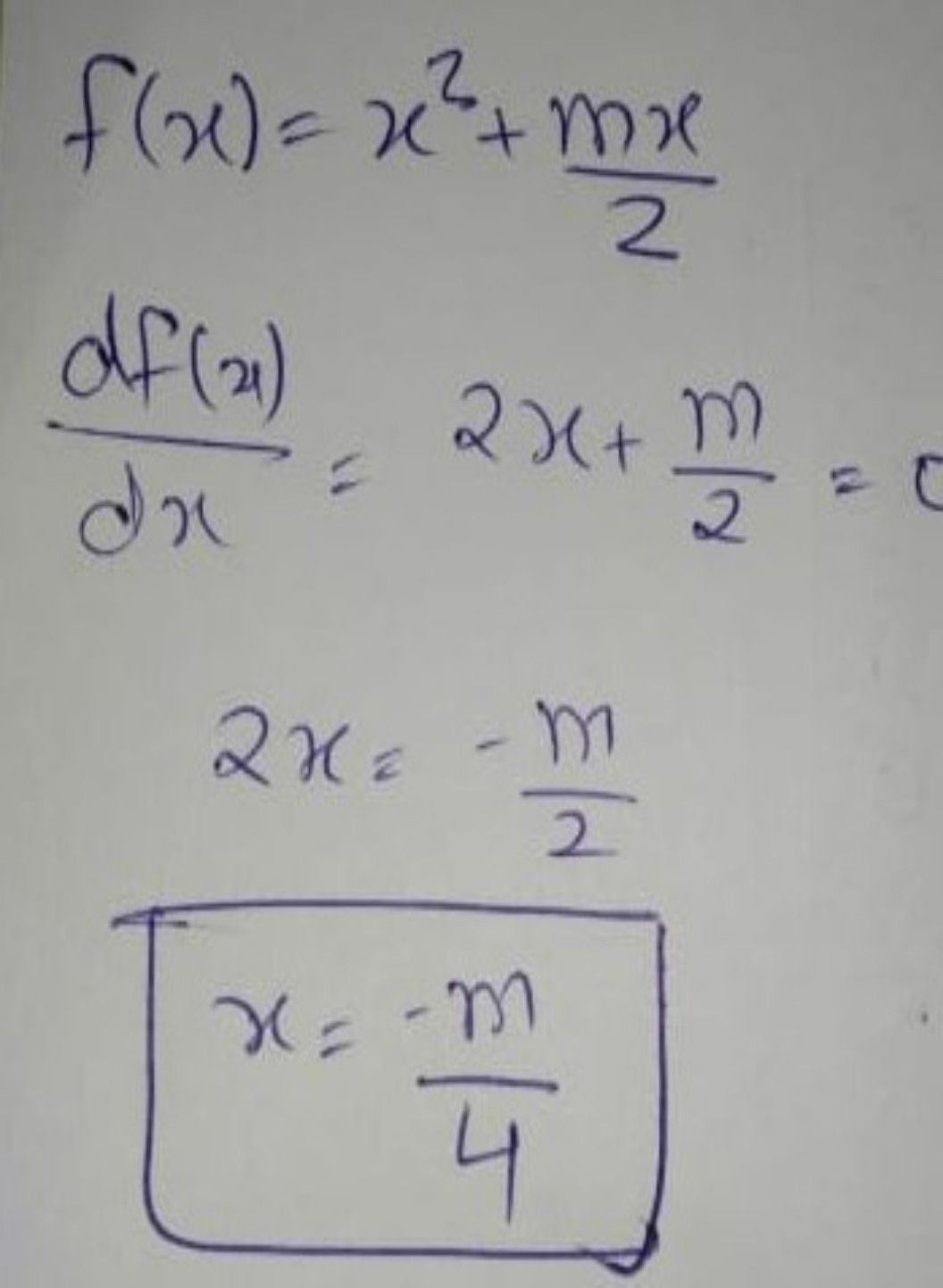Symbol
Problem#This question was automatically translated by Qanda $A1$ The proper process $t09$ obtain $m$ knowing that $ts$ local minimum $s$ $s$ $nclonf\left(x\right)=x^{n}\left(2\right)+\times \left(m\right)$ $\left(x\right)is$ Select a $=2e$ in the funclon